Provide a cell reference by taking a row and column number

## What is the Cell ADDRESS Function?

The cell ADDRESS function is categorized under Excel Lookup and Reference functions. It will provide a cell reference (its “address”) by taking the row number and column letter. The cell reference will be provided as a string of text. The function can return an address in a relative or absolute format and can be used to construct a cell reference inside a formula.

As a financial analyst, cell ADDRESS can be used to convert a column number to a letter, or vice versa. We can use the function to address the first cell or last cell in a range.

### Formula

The formula uses the following arguments:

1. Row_num (required argument) – This is a numeric value specifying the row number to be used in the cell reference.
2. Column_num (required argument) – A numeric value specifying the column number to be used in the cell reference.
3. Abs_num (optional argument) – This is a numeric value specifying the type of reference to return:

Abs_numReturns this type of reference
1 or omittedAbsolute
2Absolute row; relative column
3Absolute column; relative row
4Relative

4. A1(optional argument) – This is a logical value specifying the A1 or R1C1 reference style. In R1C1 reference style, both columns and rows are labeled numerically. It can either be TRUE (reference should be A1) or FALSE (reference should be R1C1).

When omitted, it will take on the default value TRUE (A1 style).

1. Sheet_text (optional argument) – Specifies the sheet name. If we omit the argument, it will take the current worksheet.

### How to use the ADDRESS Function in Excel?

To understand the uses of the cell ADDRESS function, let us consider a few examples:

#### Example 1

Suppose we wish to convert the following numbers into Excel column references: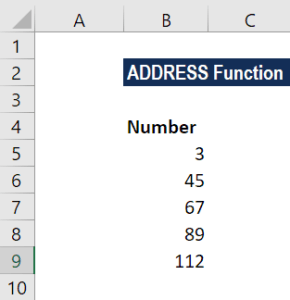The formula to use will be: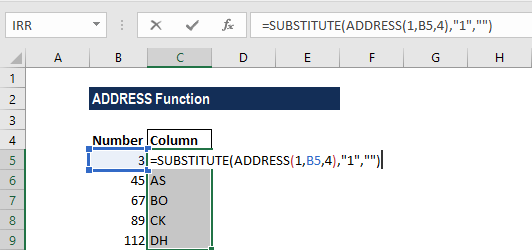We get the results below: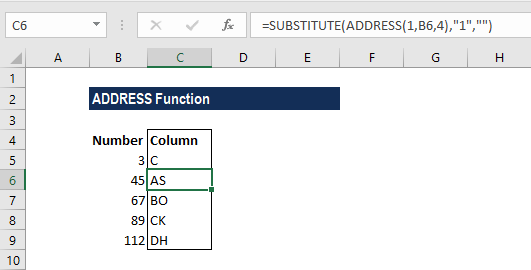The ADDRESS function will first construct an address containing the column number. It was done by providing 1 for row number, a column number from B6, and 4 for the abs_num argument.

After that, we use the SUBSTITUTE function to take out the number 1 and replace with “”.

#### Example 2

The ADDRESS function can be used to convert a column letter to a regular number, e.g., 21, 100, 126, etc. We can use a formula based on the INDIRECT and COLUMN functions.

Suppose we are given the following data: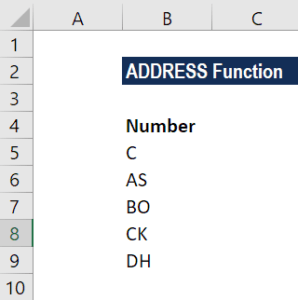The formula to use will be: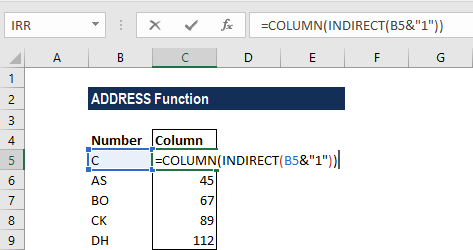We get the results below: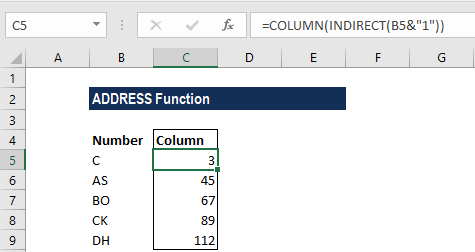The INDIRECT function transforms the text into a proper Excel reference and hands the result off to the COLUMN function. Then, the COLUMN function evaluates the reference and returns the column number for the reference.

1. If we wish to change the reference style that Excel uses, we should go to the File tab, click Options, and then select Formulas. Under Working with formulas, we can select or clear the R1C1 reference style checkbox.
2. #VALUE! error – Occurs when any of the arguments are invalid. We would get this argument if:
• The row_num is less than 1 or greater than the number of rows in the spreadsheet;
• The column_num is less than 1 or greater than the number of columns in the spreadsheet; or
• Any of the supplied row_num, column_num or [abs_num] arguments are non-numeric or the supplied [a1] argument is not recognized as a logical value.

Thanks for reading CFI’s guide to important Excel functions! By taking the time to learn and master these functions, you’ll significantly speed up your financial analysis. To learn more, check out these additional CFI resources:

• Excel Functions for Finance